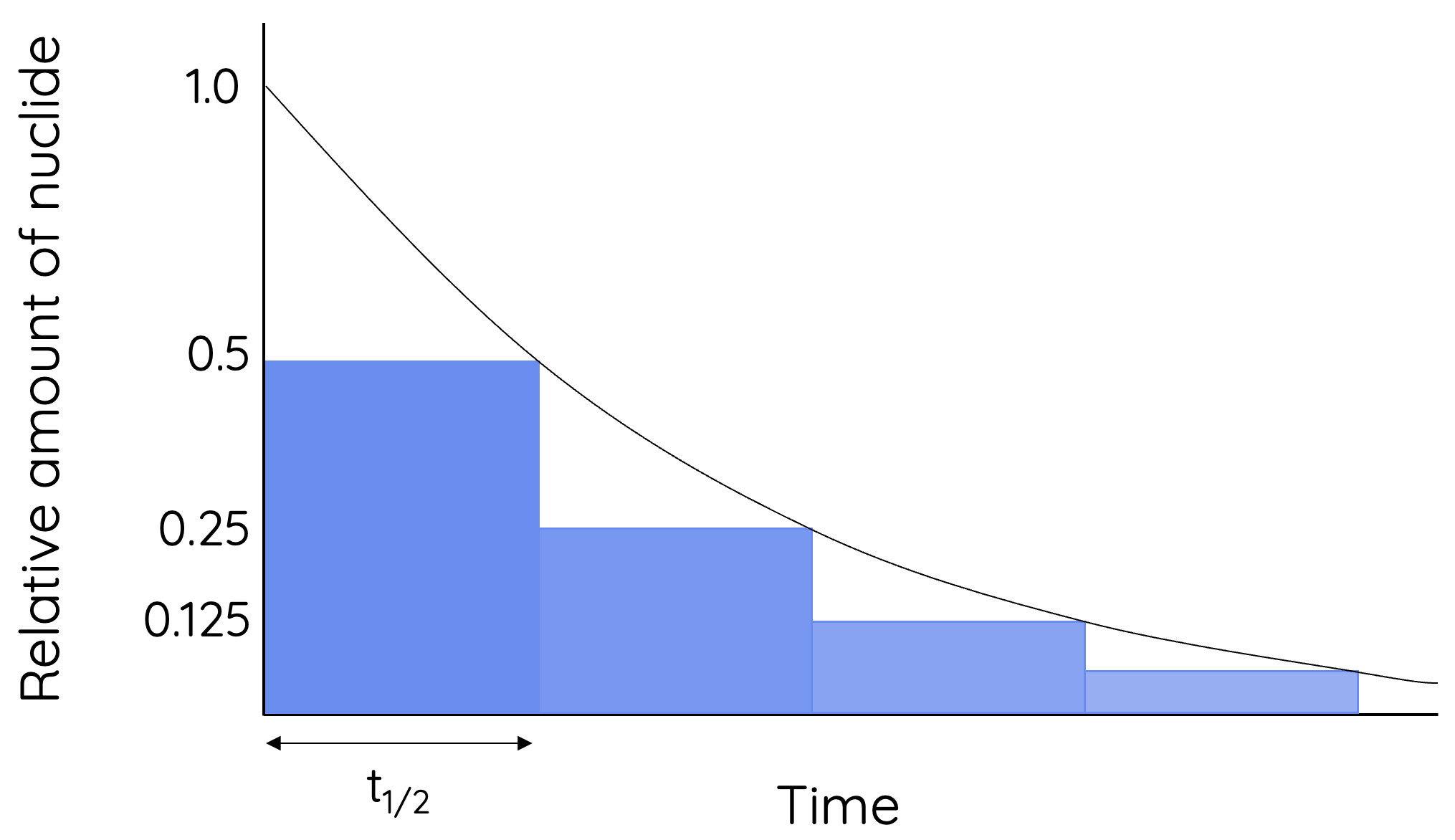This topic is part of the HSC Physics course under the section Properties of the Nucleus.

### HSC Physics Syllabus

• examine the model of half-life in radioactive decay and make quantitative predictions about the activity or amount of a radioactive sample using the following relationships:
– N_t = N_0 e^{-\lambda t}
– \lambda = \frac{ln(2)}{t_{\frac{1}{2}}}

where N_t = number of particles at time t, N_0 = number of particles present at t = 0,  \lambda = decay constant, t_{1/2} =  time for half the radioactive amount to decay (ACSPH029)

Radioactive decay can be modelled by the equation:

$$N_t=N_0e^{-\lambda t}$$

where Nt is the number of nuclides present after time t. N0 is the initial number of nuclides when t = 0. \lambda is the decay constant which is specific for a particular isotope.

The equation above suggests that the rate of decay decreases with time. The rate of radioactive decay is always the greatest at the beginning.

### What is Half-life?

Half-life of a radioisotope is the time taken for half the amount of the parent nuclide to decay into the daughter nuclide.

This occurs when:Where t1/2 is the half-life which stays constant throughout a nuclide’s decay process. However, the amount of nuclide disintegrated during each interval of half-life decreases throughout the process (see below).Figure shows the change in radioactive nuclide over time as modelled by exponential decay. For every half-life interval the amount of parent nuclide is halved - hence the term 'half-life'.

For example, a particular radioisotope will take t1/2 for 50% of its original quantity (No) to disintegrate. It will take 2t1/2 for 25%, 3t1/2 for 12.5% and so on.

### What is Decay Constant?

Decay constant of a radioisotope describes the number of nuclide that decays per unit time. A larger decay constant suggests a radioisotope decays faster.As shown by the derivation above, the decay constant is given by

$$\lambda = \frac{ln(2)}{t_{\frac{1}{2}}}$$

The decay constant is inversely proportional to a nuclide's half-life. A larger decay constant means a nuclide takes shorter time to reach 50% of its initial quantity, and therefore has a shorter half-life.

As parent nuclides decay, the quantity of daughter nuclides increases. The increase in daughter nuclides is modelled logarithmically.What units are suitable for radioactive decay calculation?

Normally, SI units are always encouraged for performing calculations in physics. However, there is a degree of flexibility when performing calculations for radioactive decay.

Half-life or time can be provided in various units such as hours and years. They do not need to be converted into seconds. The unit you use depends on the unit of the decay constant. Conversely, when calculating the decay constant, its unit depends on the unit of the half-life.

For example, if the decay constant has a unit of yr-1 then time values in the unit of year can be used in the same equation.

 Unit for time (t) Unit for decay constant (𝜆) second s–1 minute min–1 hour hr–1 year yr–1

The half-life of cobalt-60 nuclide is 5.30 years.The mass of a particular sample of cobalt-60 was 100 kg.

Calculate the mass of this sample after 12 years.

Solution:

First, calculate the decay constant for Co-60:

$$\lambda = \frac{ln(2}{t_{\frac{1}{2}}}$$

$$\lambda = \frac{ln(2)}{5.30}$$

$$\lambda = 0.131 \hspace{0.1cm} year^{-1}$$

Use decay equation:

$$N_t=N_0e^{-\lambda t}$$

$$N_t=(100)e^{-(0.131)(12)}$$

$$N_t=20.8 \hspace{0.1cm} kg$$